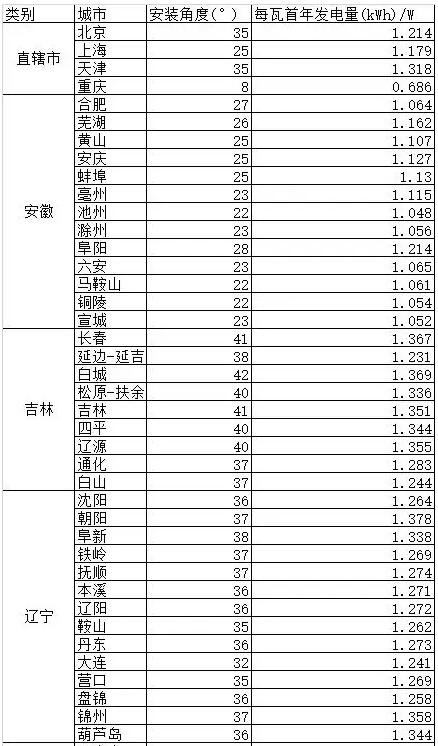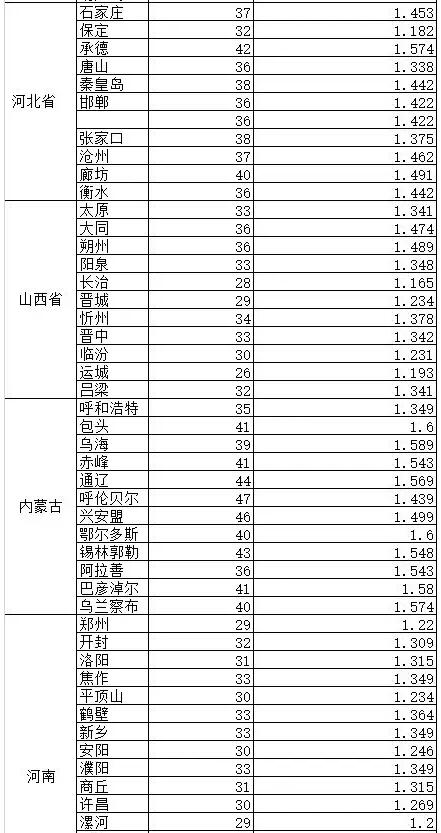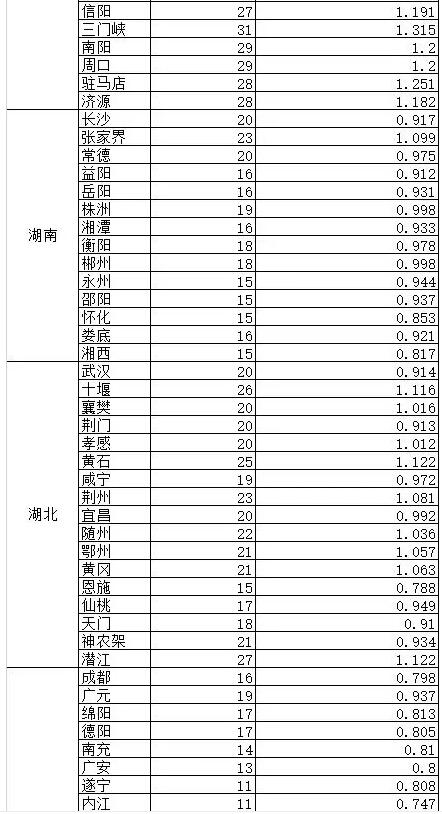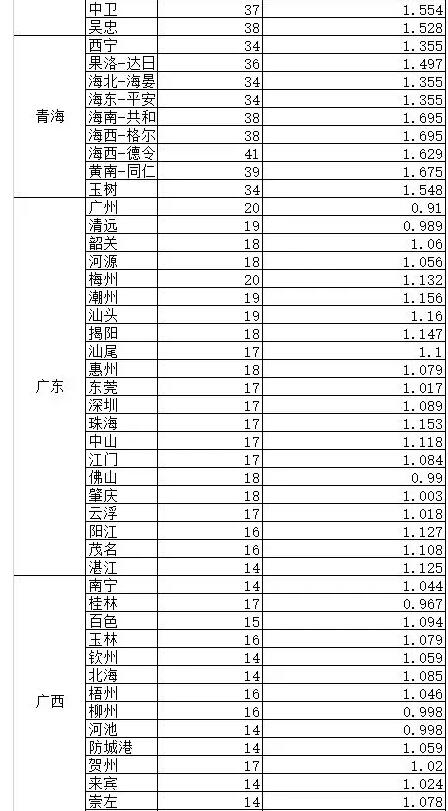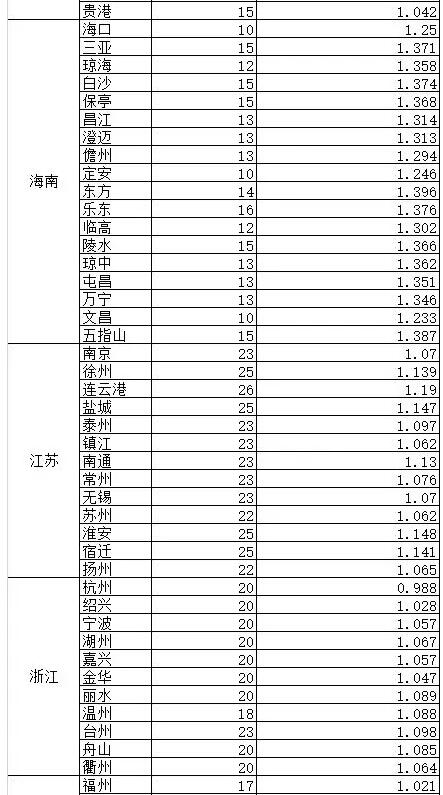﻿光伏逆变器厂家教你如何选择光伏系统|深圳市美克能源科技股份有限公司

## 新闻中心

### 光伏逆变器厂家教你如何选择光伏系统

2018-3-13

“我想装一个光伏电站，但是不知道到底能赚多钱，能帮我算一下么?”

“我想装一个光伏电站，几年能回本啊?”

“为什么有的户用光伏是5.8元/瓦，有的是6.3元/瓦，我该怎么选择啊?”

…………

Σ=W*H*B*20+B′*W*H*0.8*5+W*H*T*N-W*Z-12*S*25*M

W=装机容量(1KW=1000W)

H=单瓦首年发电量(瓦/千瓦时)

B=当地的光伏标杆上网电价

B′=当地脱硫煤标杆电价

T=当地省市补贴情况

N=当地省市补贴期限

Z=系统单瓦造价

S=月用电量

M=民用电价格

W=8 KW(8000瓦)

H=1.341(瓦/kWh)

B=0.75(元/度)

B′=0.332(元/度)

T=0(元/度)

N=0(年)

Z=6(元)

S=200(kWh)

M=0.5(元/kWh)

Σ=8000*1.341*0.75*20+8000*0.332*1.341*0.8*5+8000*1.341*0*0-8000*6-12*200*25*0.5

=160920+14246.784+0-48000-30000=97166.784(元)

W*Z/(B*W*H-12*S*M)

Δ=8000*6/(0.75*8000*1.341-12*200*0.5)

=7.011年

Σ=[W*H*0.37+12*S*M+(W*H-12*S)*B′]*20+W*H*T*N+(W*H*0.8-12*S)*B′*5+12*S*M*5-W*Z

Σ=[8000*1.341*0.37+12*200*0.5+(8000*1.341-12*200)*0.332]*20+8000*1.341*0*0+(8000*1.341*0.8-12*200)*0.332*5+12*200*0.5*5-8000*6

=[3969.36+1200+2764.896]*20+0+10262.784+6000-48000

=126947.904(元)

W*Z/[W*H*0.37+12*S*M+(W*H-12*S)*B′+W*H*T*N]

Δ=8000*6/[8000*1.341*0.37+12*200*0.5+(8000*1.341-12*200)*0.332+8000*1.341*0*0]=6.05年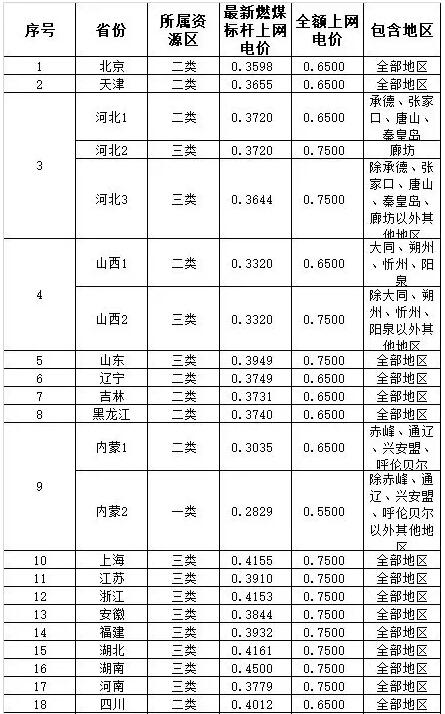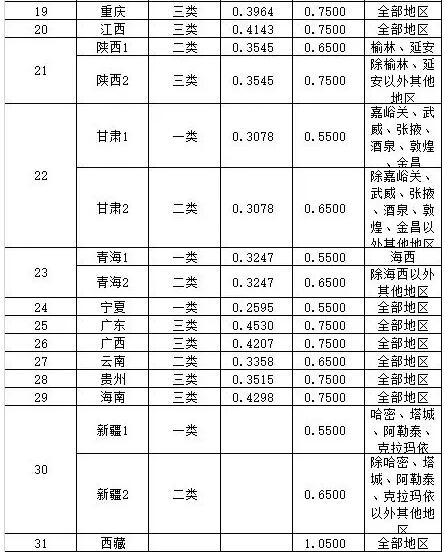附表2